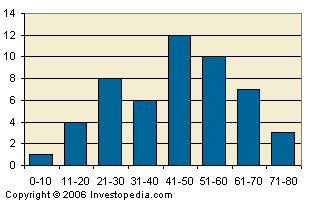# Histogram

Histogram
1. A graphical representation, similar to a bar chart in structure, that organizes a group of data points into user-specified ranges. The histogram condenses a data series into an easily interpreted visual by taking many data points and grouping them into logical ranges or bins.2. The MACD histogram is a very common technical indicator that illustrates the difference between the MACD line and the trigger line. This difference is then plotted on a chart in the form of a histogram to make it easy for a trader to determine a specific asset's momentum.

1. Histograms are commonly used in statistics to demonstrate how many of a certain type of variable occurs within a specific range. For example, a census focused on the demography of a country may use a histogram of how many people there are between the ages of 0 and 10, 11 and 20, 21 and 30, 31 and 40, 41 and 50 etc. This histogram would look similar to the graph above.

2. MACD histograms are a popular tool used in technical analysis to gauge the strength of an asset's momentum. An increasing MACD histogram signals an increase in upward momentum while a decreasing histogram is used to signal downward momentum.

Investment dictionary. . 2012.

### Look at other dictionaries:

• histogram — 1891, from HISTO (Cf. histo ) + GRAM (Cf. gram) …   Etymology dictionary

• histogram — histògram m DEFINICIJA grafički prikaz distribucije frekvencija gdje se frekvencije niza predočuju pravokutnicima ETIMOLOGIJA histo + gram …   Hrvatski jezični portal

• histogram — [his′tə gram΄] n. [ HISTO(RY) + GRAM] Statistics a bar graph in which the area of each bar is proportional to the frequency or relative frequency represented …   English World dictionary

• Histogram — In statistics, a histogram is a graphical display of tabulated frequencies, shown as bars. It shows what proportion of cases fall into each of several categories. A histogram differs from a bar chart in that it is the area of the bar that denotes …   Wikipedia

• histogram — A table or bar chart displaying a probability distribution. All of the probabilities in the histogram total to 100 percent. The frequency of the data for each interval is represented by the height of the bar. The technique can be used to combine… …   Financial and business terms

• histogram — /his teuh gram /, n. Statistics. a graph of a frequency distribution in which rectangles with bases on the horizontal axis are given widths equal to the class intervals and heights equal to the corresponding frequencies. [1890 95; HISTO + GRAM1]… …   Universalium

• histogram — UK [ˈhɪstəɡræm] / US [ˈhɪstəˌɡræm] noun [countable] Word forms histogram : singular histogram plural histograms maths a type of bar chart that shows a numerical value on each axis …   English dictionary

• histogram — 1. noun A graphical display of numerical data in the form of upright bars, with the area of each bar representing frequency. 2. verb To represent (data) as a histogram …   Wiktionary

• histogram — s ( met, histogram, best. pl. men) typ av diagram …   Clue 9 Svensk Ordbok

• histogram — histograma statusas T sritis biomedicinos mokslai apibrėžtis ↑Kintamojo reikšmių, grupuotų intervalais, skirstymo grafiškas vaizdavimas stulpeline diagrama. Stulpelio plotis yra proporcingas kintamojo reikšmių intervalui, o aukštis – šių reikšmių …   Lithuanian dictionary (lietuvių žodynas)